# Monopoly o A firm is considered a monopoly

• Slides: 82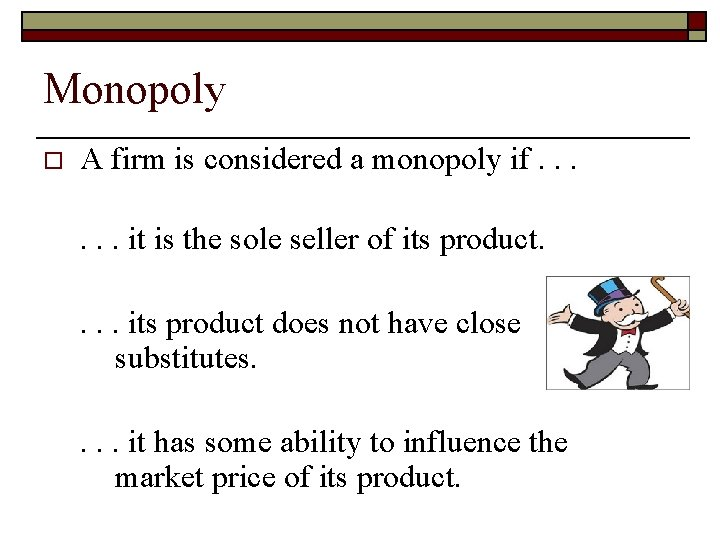Monopoly o A firm is considered a monopoly if. . . it is the sole seller of its product. . its product does not have close substitutes. . it has some ability to influence the market price of its product.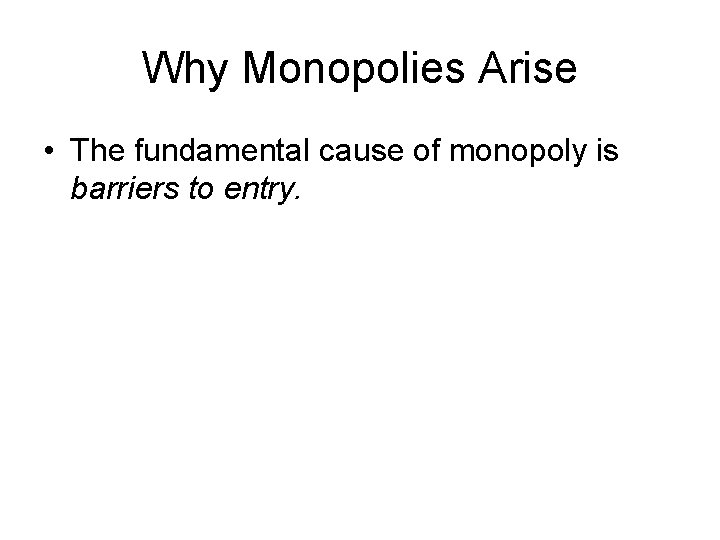Why Monopolies Arise • The fundamental cause of monopoly is barriers to entry.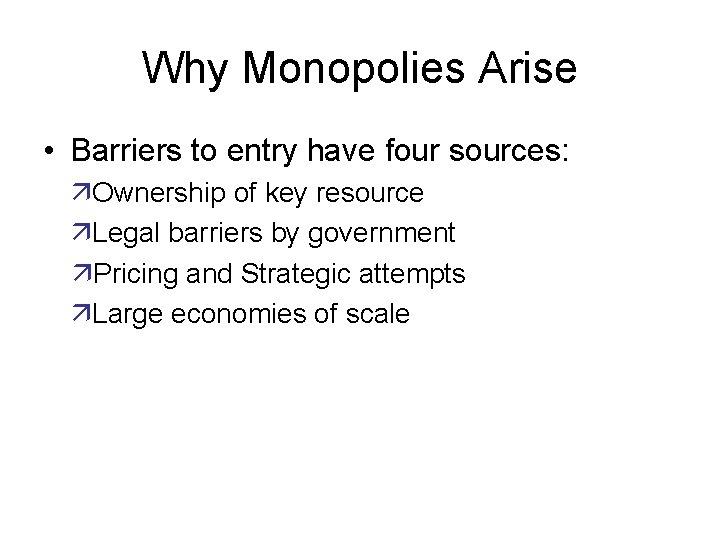Why Monopolies Arise • Barriers to entry have four sources: äOwnership of key resource äLegal barriers by government äPricing and Strategic attempts äLarge economies of scale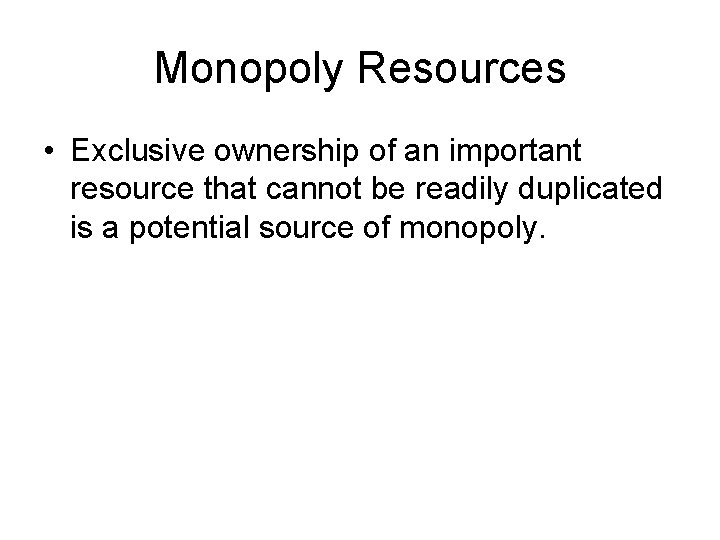Monopoly Resources • Exclusive ownership of an important resource that cannot be readily duplicated is a potential source of monopoly.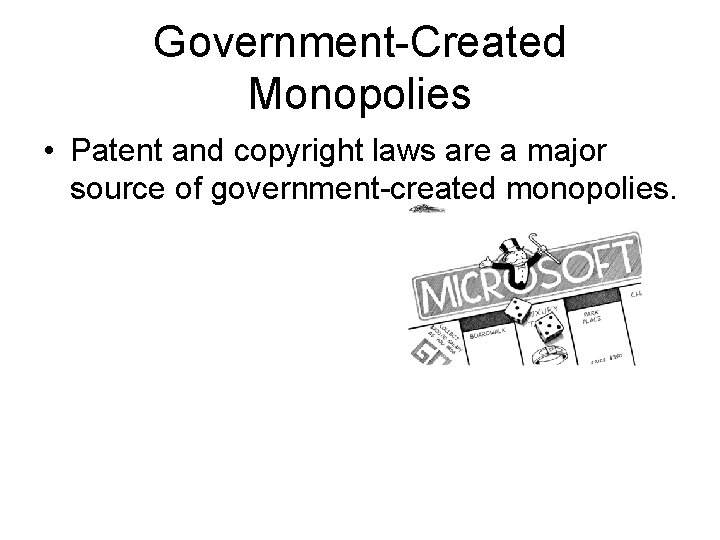Government-Created Monopolies • Patent and copyright laws are a major source of government-created monopolies.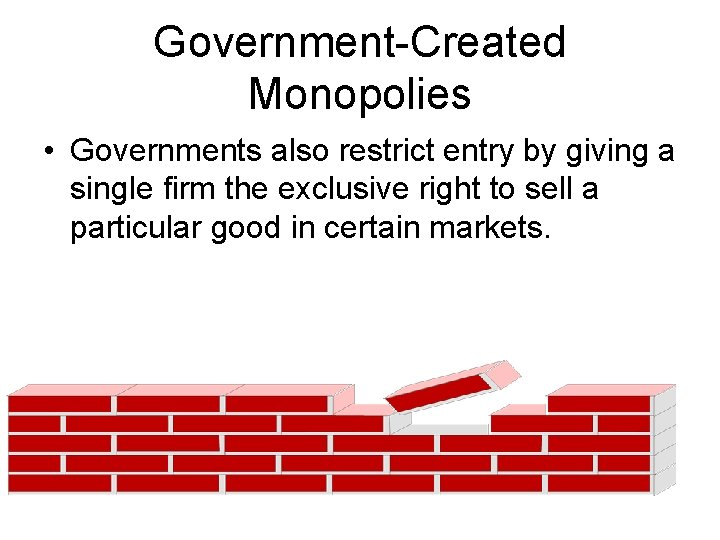Government-Created Monopolies • Governments also restrict entry by giving a single firm the exclusive right to sell a particular good in certain markets.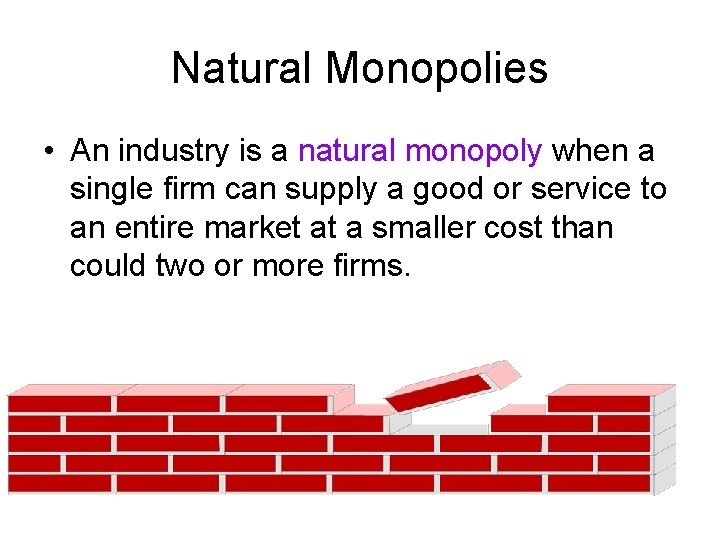Natural Monopolies • An industry is a natural monopoly when a single firm can supply a good or service to an entire market at a smaller cost than could two or more firms.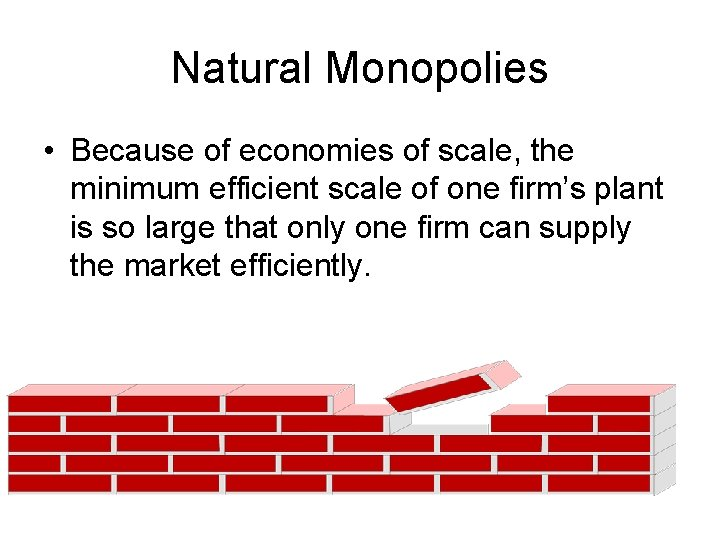Natural Monopolies • Because of economies of scale, the minimum efficient scale of one firm’s plant is so large that only one firm can supply the market efficiently.Quick Quiz! • What are three reasons that a market might have a monopoly?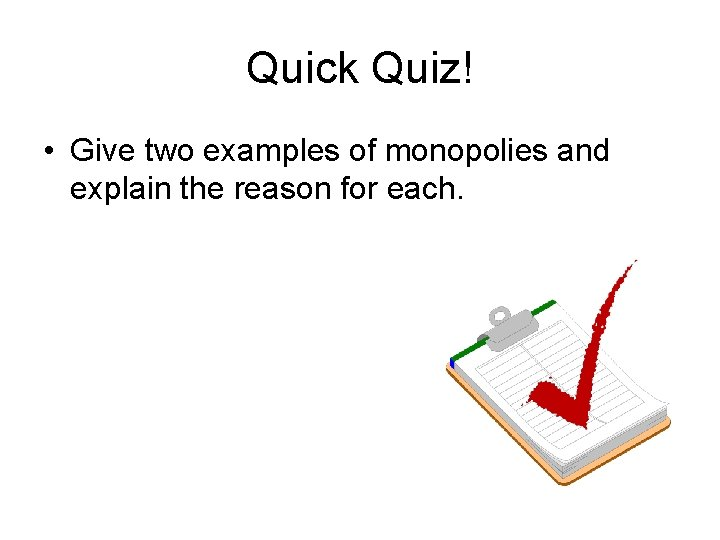Quick Quiz! • Give two examples of monopolies and explain the reason for each.Monopoly versus Competition • Monopoly äIs the sole producer äIs a price maker äHas a downward-sloping demand curve • reduces price to increase salesCompetition versus Monopoly • Competitive Firm äIs one of many producers äIs a price taker äHas a horizontal demand curve • Sells as much or as little at same price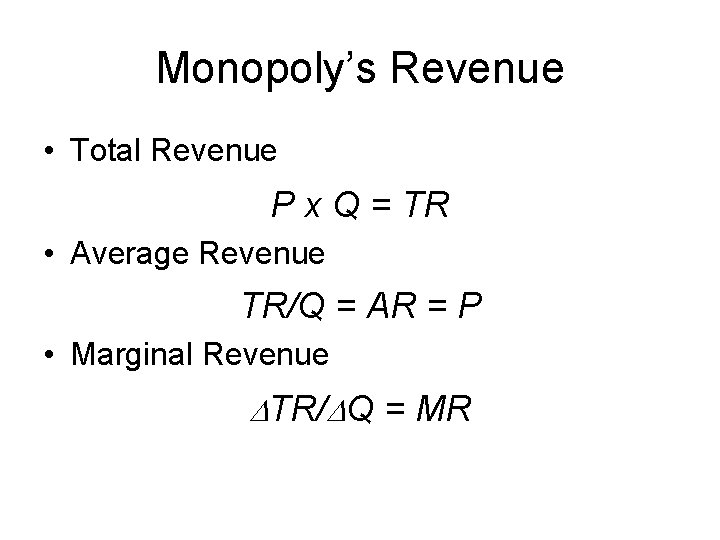Monopoly’s Revenue • Total Revenue P x Q = TR • Average Revenue TR/Q = AR = P • Marginal Revenue DTR/DQ = MR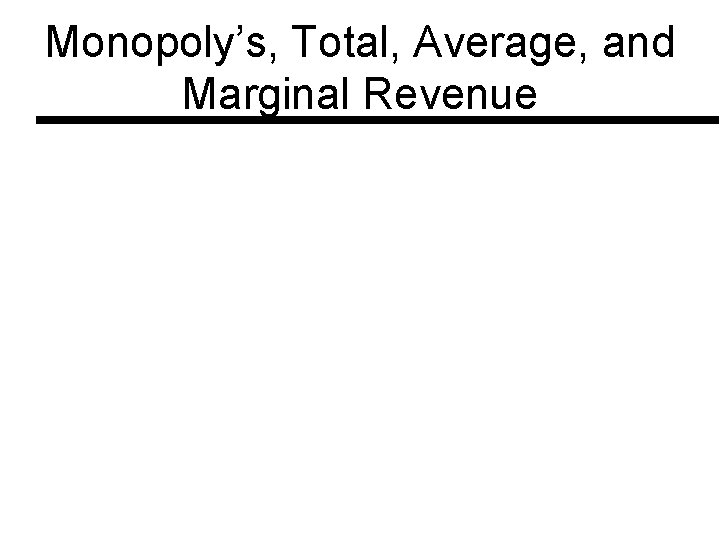Monopoly’s, Total, Average, and Marginal Revenue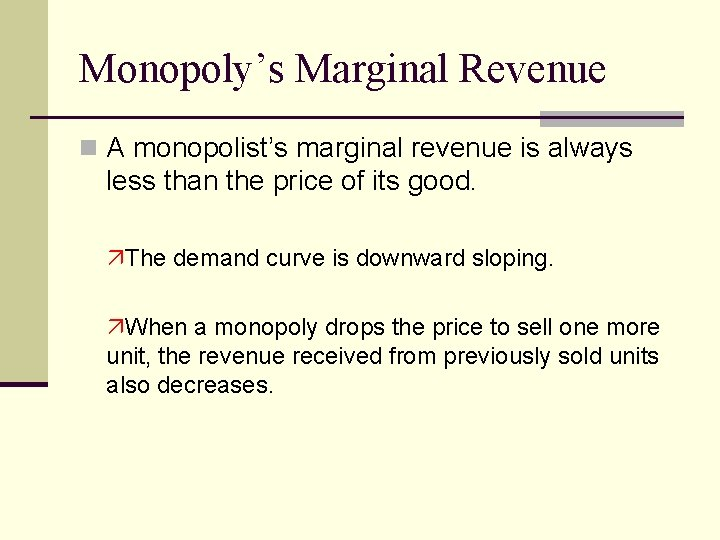Monopoly’s Marginal Revenue n A monopolist’s marginal revenue is always less than the price of its good. äThe demand curve is downward sloping. äWhen a monopoly drops the price to sell one more unit, the revenue received from previously sold units also decreases.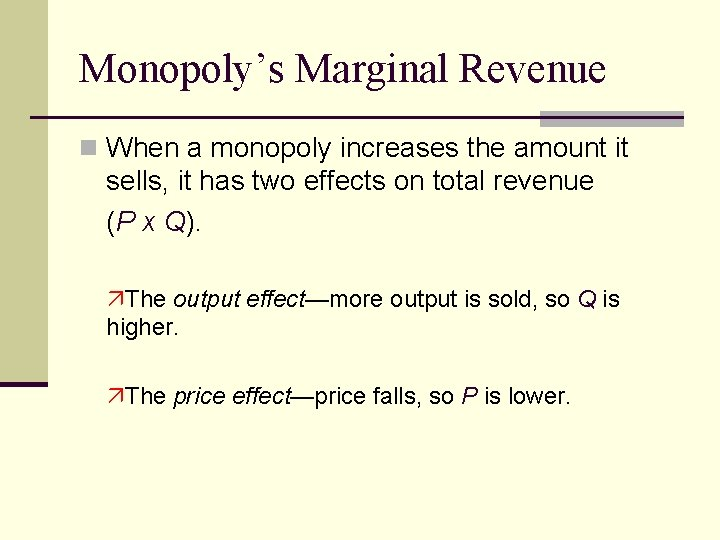Monopoly’s Marginal Revenue n When a monopoly increases the amount it sells, it has two effects on total revenue (P x Q). äThe output effect—more output is sold, so Q is higher. äThe price effect—price falls, so P is lower.Monopoly’s Marginal Revenue n The marginal revenue curve lies below its demand curve.Monopoly’s Demand Marginal Revenue Curves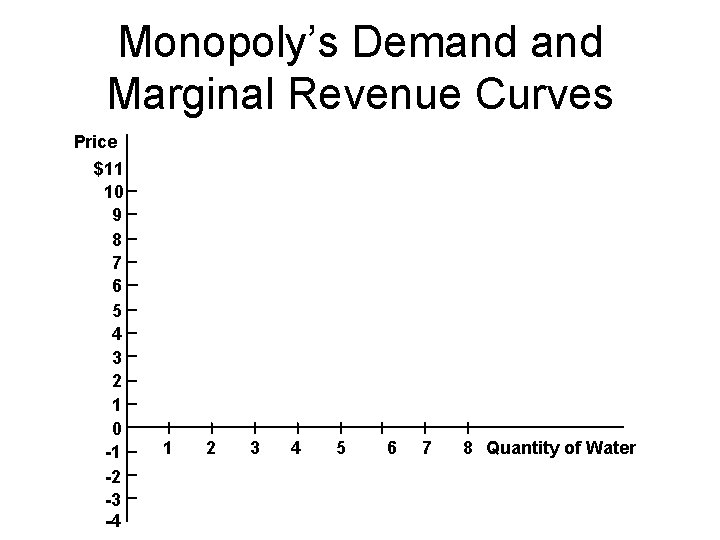Monopoly’s Demand Marginal Revenue Curves Price \$11 10 9 8 7 6 5 4 3 2 1 0 -1 -2 -3 -4 1 2 3 4 5 6 7 8 Quantity of Water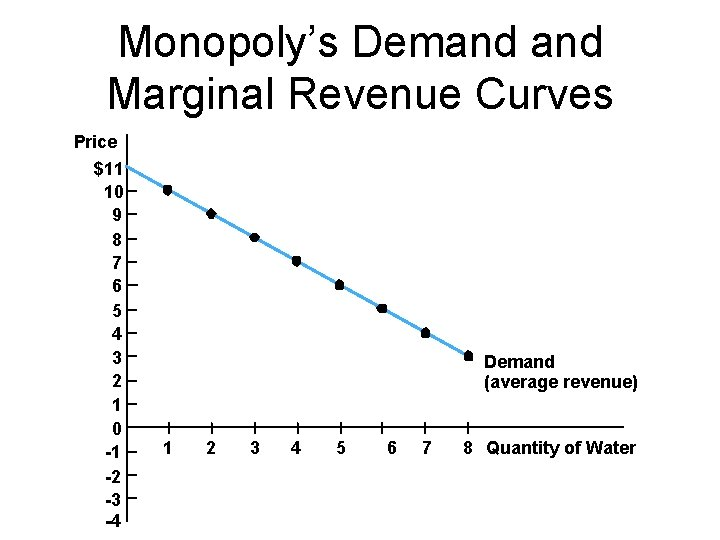Monopoly’s Demand Marginal Revenue Curves Price \$11 10 9 8 7 6 5 4 3 2 1 0 -1 -2 -3 -4 Demand (average revenue) 1 2 3 4 5 6 7 8 Quantity of Water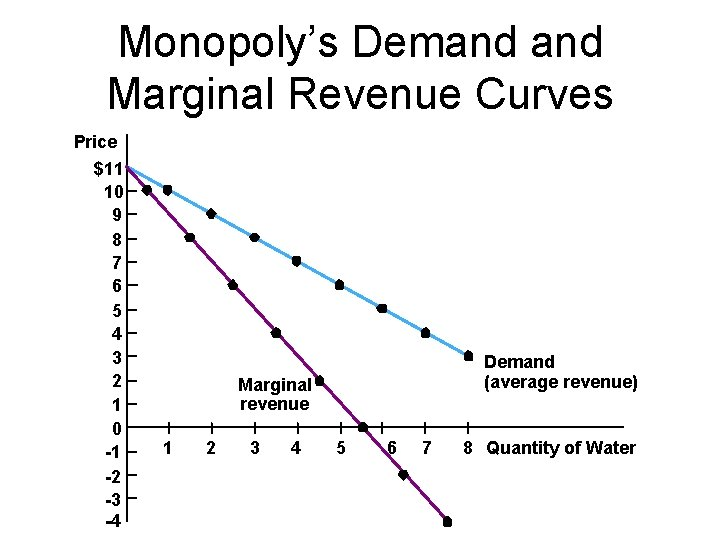Monopoly’s Demand Marginal Revenue Curves Price \$11 10 9 8 7 6 5 4 3 2 1 0 -1 -2 -3 -4 Demand (average revenue) Marginal revenue 1 2 3 4 5 6 7 8 Quantity of Water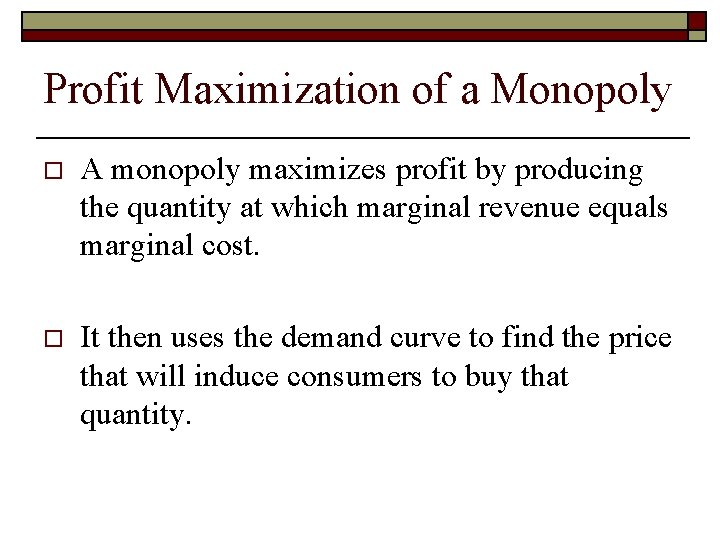Profit Maximization of a Monopoly o A monopoly maximizes profit by producing the quantity at which marginal revenue equals marginal cost. o It then uses the demand curve to find the price that will induce consumers to buy that quantity.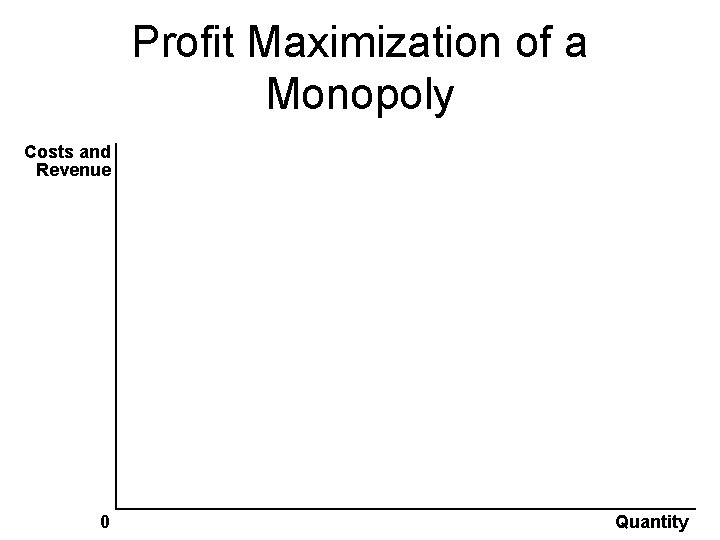Profit Maximization of a Monopoly Costs and Revenue 0 QuantityProfit Maximization of a Monopoly Costs and Revenue Demand Marginal revenue 0 QuantityProfit Maximization of a Monopoly Costs and Revenue Marginal cost Average total cost Demand Marginal revenue 0 QuantityProfit Maximization of a Monopoly Costs and Revenue Marginal cost Average total cost A Demand Marginal revenue 0 Quantity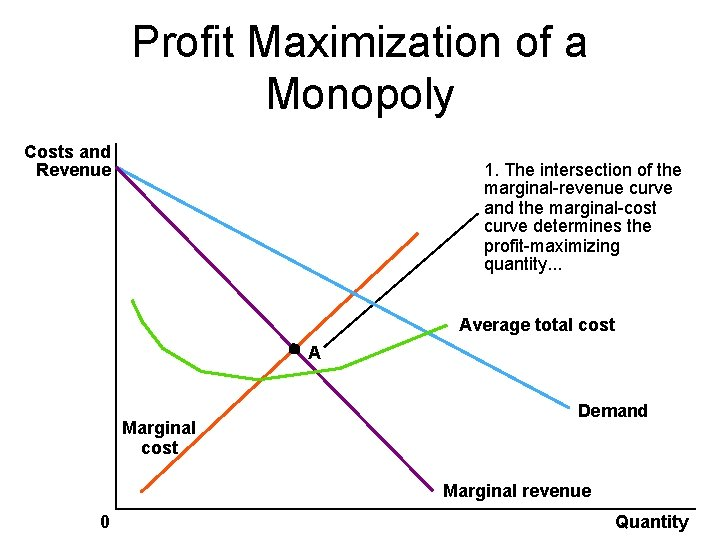Profit Maximization of a Monopoly Costs and Revenue 1. The intersection of the marginal-revenue curve and the marginal-cost curve determines the profit-maximizing quantity. . . Average total cost A Marginal cost Demand Marginal revenue 0 Quantity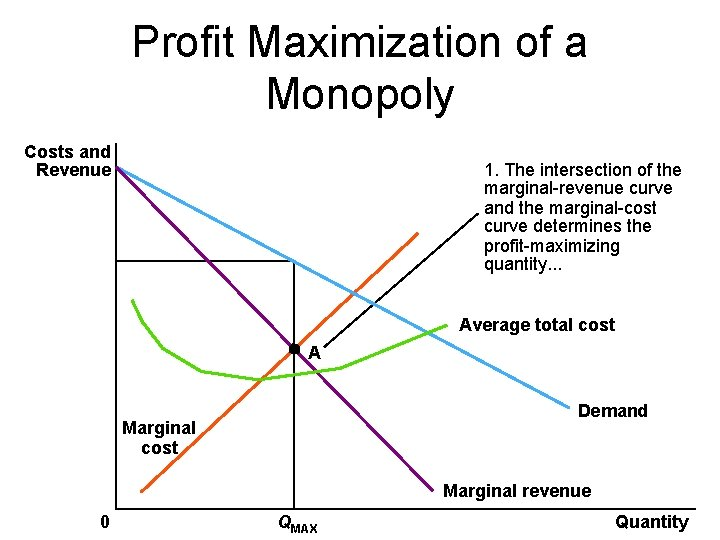Profit Maximization of a Monopoly Costs and Revenue 1. The intersection of the marginal-revenue curve and the marginal-cost curve determines the profit-maximizing quantity. . . Average total cost A Demand Marginal cost Marginal revenue 0 QMAX QuantityProfit Maximization of a Monopoly Costs and Revenue 2. . and then the demand curve shows the price consistent with this quantity. B Monopoly price 1. The intersection of the marginal-revenue curve and the marginal-cost curve determines the profit-maximizing quantity. . . Average total cost A Demand Marginal cost Marginal revenue 0 QMAX Quantity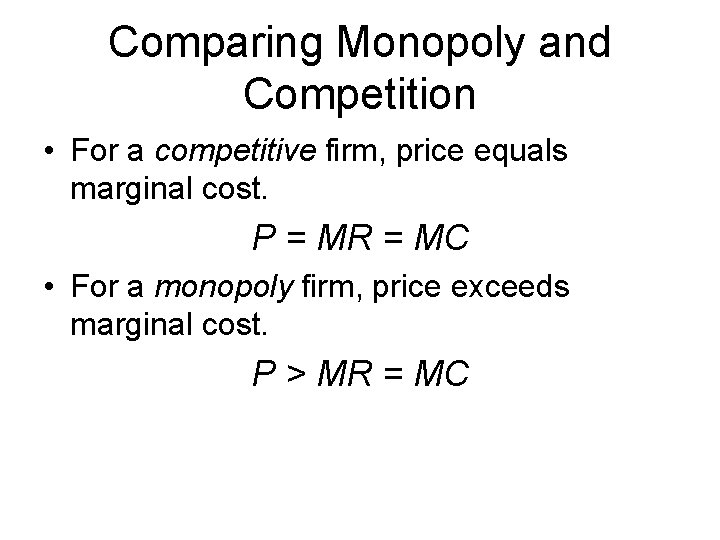Comparing Monopoly and Competition • For a competitive firm, price equals marginal cost. P = MR = MC • For a monopoly firm, price exceeds marginal cost. P > MR = MCCalculating Monopoly Profit • Profit equals total revenue minus total costs. Profit = TR - TC Profit = (TR/Q - TC/Q) x Q Profit = (P - ATC) x Q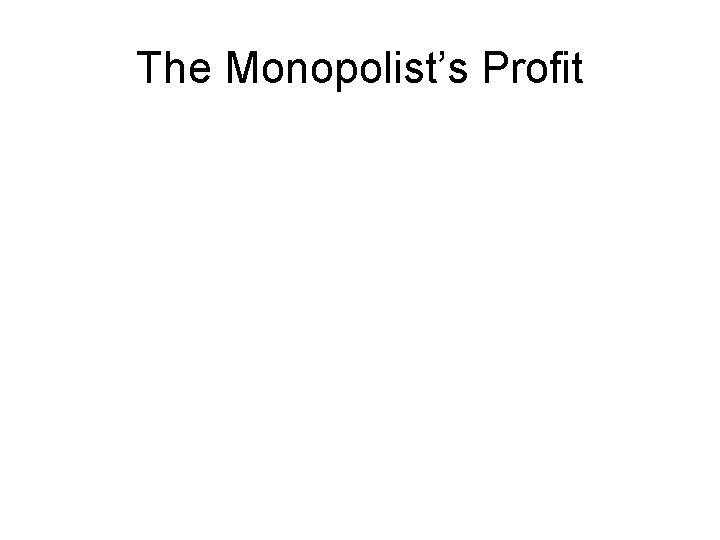The Monopolist’s Profit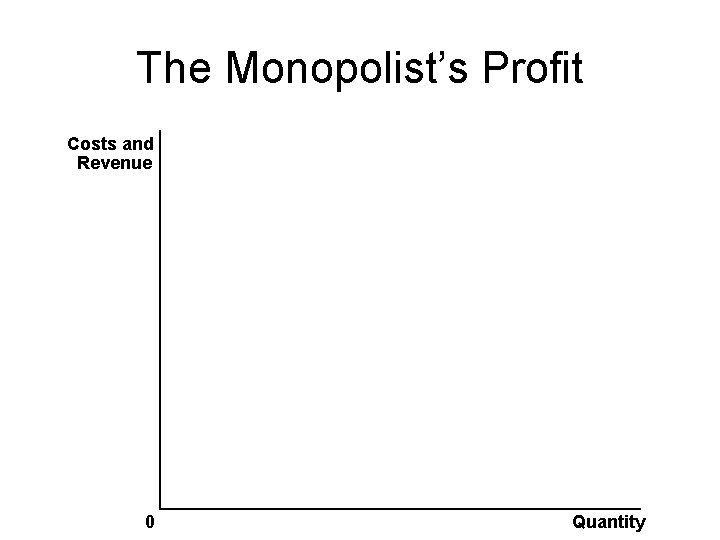The Monopolist’s Profit Costs and Revenue 0 QuantityThe Monopolist’s Profit Costs and Revenue Demand Marginal revenue 0 Quantity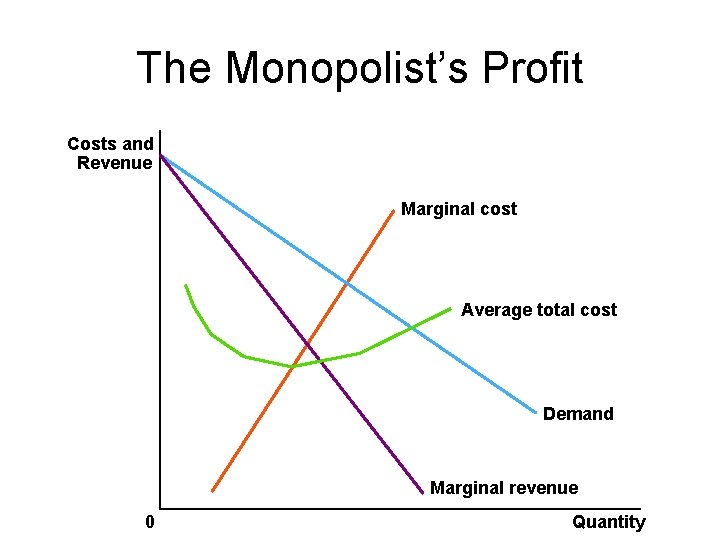The Monopolist’s Profit Costs and Revenue Marginal cost Average total cost Demand Marginal revenue 0 Quantity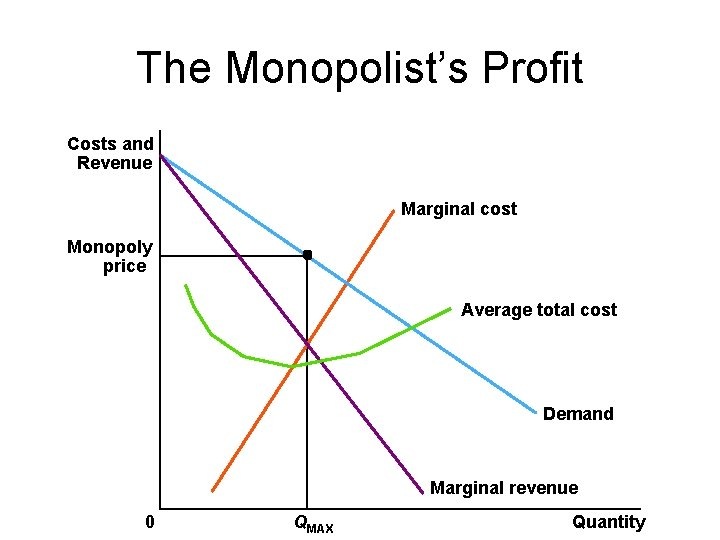The Monopolist’s Profit Costs and Revenue Marginal cost Monopoly price Average total cost Demand Marginal revenue 0 QMAX Quantity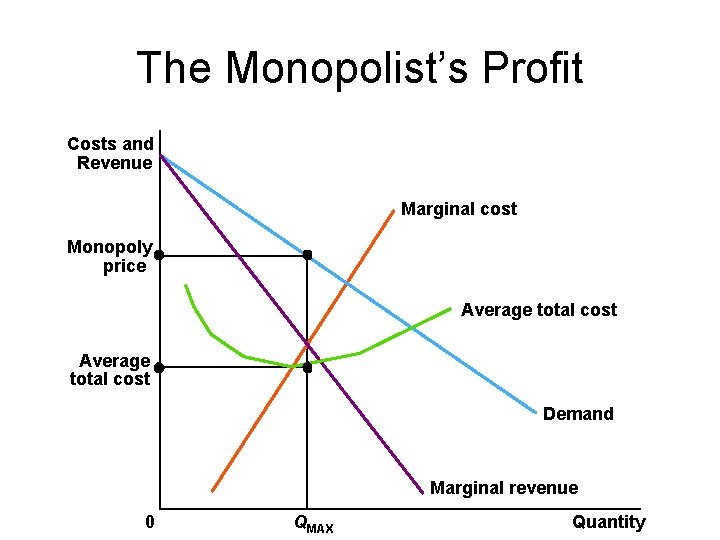The Monopolist’s Profit Costs and Revenue Marginal cost Monopoly price Average total cost Demand Marginal revenue 0 QMAX QuantityThe Monopolist’s Profit Costs and Revenue Marginal cost Monopoly E price B Monopoly prophat Average total cost D Average total cost C Demand Marginal revenue 0 QMAX Quantity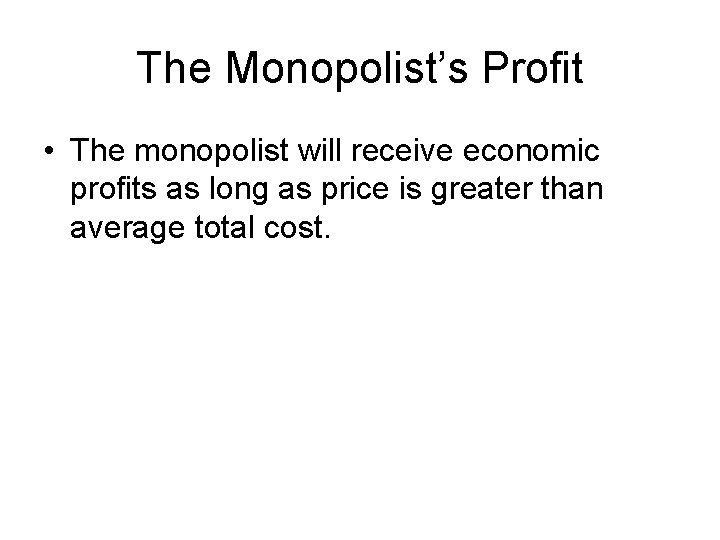The Monopolist’s Profit • The monopolist will receive economic profits as long as price is greater than average total cost.Quick Quiz! • Explain how a monopolist chooses the quantity of output to produce and the price to charge.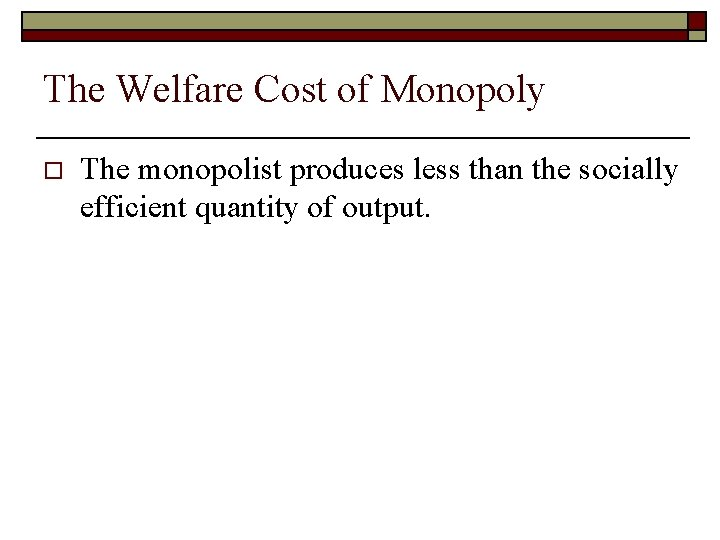The Welfare Cost of Monopoly o The monopolist produces less than the socially efficient quantity of output.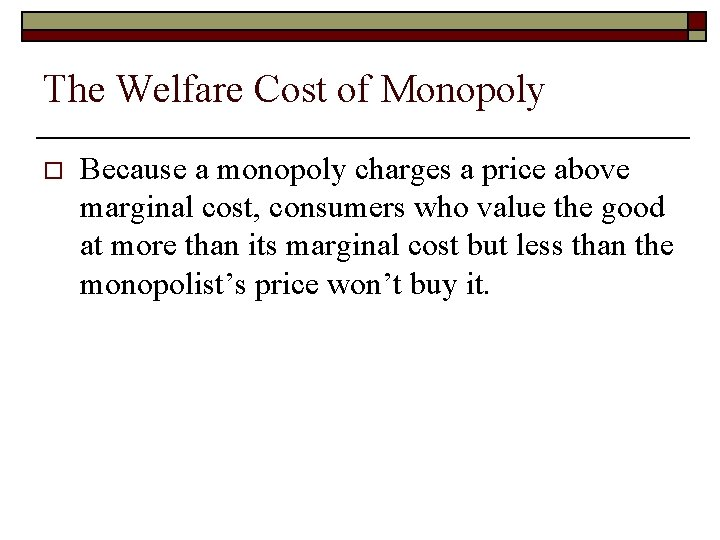The Welfare Cost of Monopoly o Because a monopoly charges a price above marginal cost, consumers who value the good at more than its marginal cost but less than the monopolist’s price won’t buy it.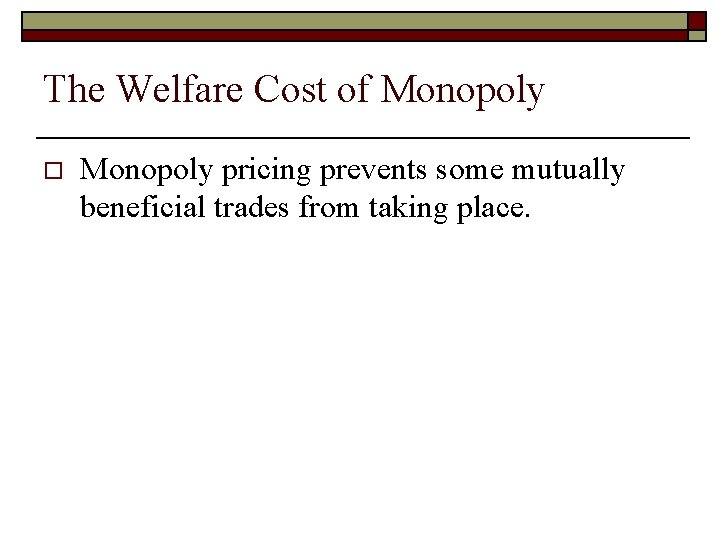The Welfare Cost of Monopoly o Monopoly pricing prevents some mutually beneficial trades from taking place.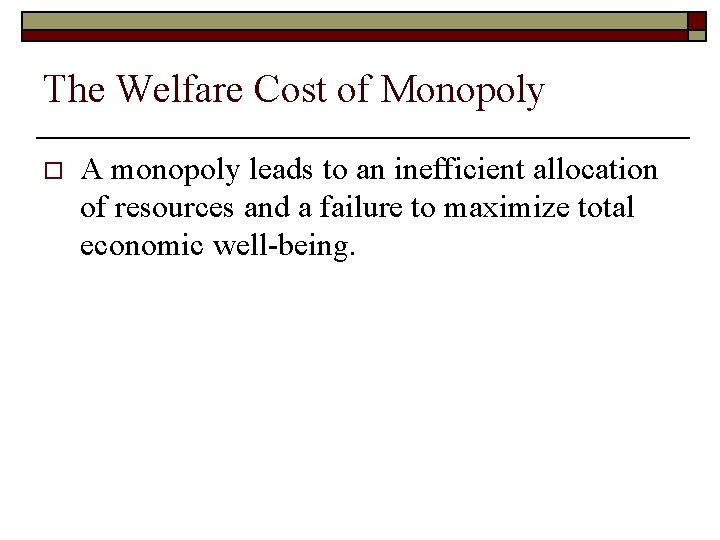The Welfare Cost of Monopoly o A monopoly leads to an inefficient allocation of resources and a failure to maximize total economic well-being.The Deadweight Loss o o Because a monopoly sets its price above marginal cost, it places a wedge between the consumer’s willingness to pay and the producer’s cost. äThis wedge causes the quantity sold to fall short of the social optimum.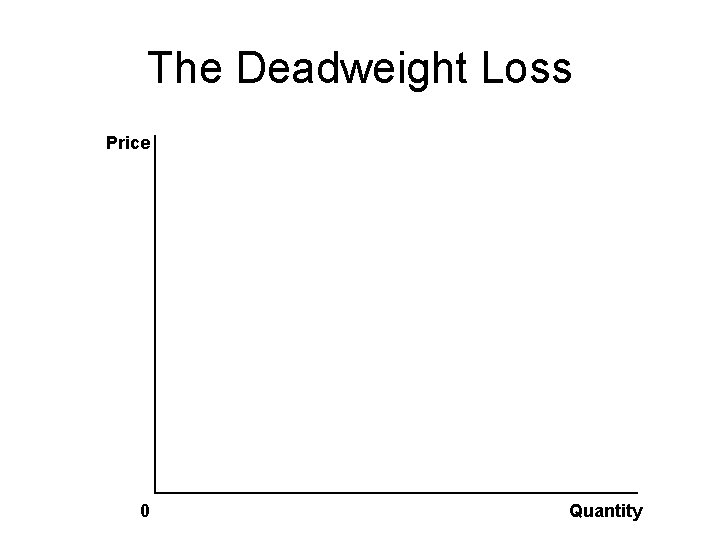The Deadweight Loss Price 0 QuantityThe Deadweight Loss Price Marginal revenue 0 Demand Quantity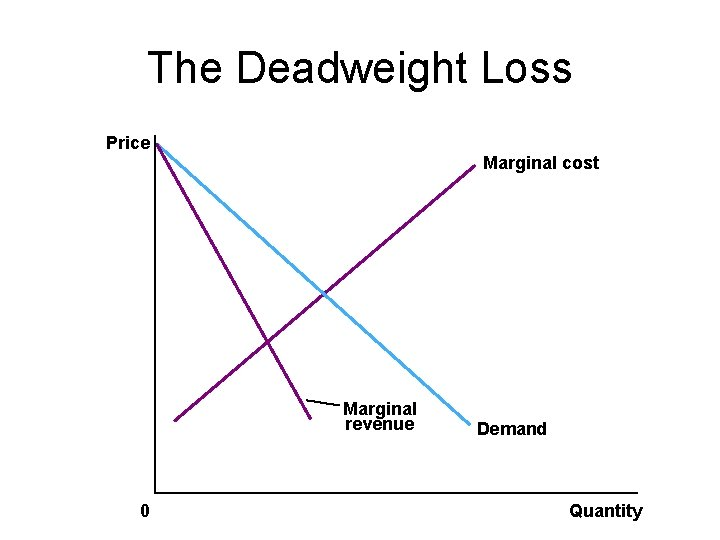The Deadweight Loss Price Marginal cost Marginal revenue 0 Demand QuantityThe Deadweight Loss Price Marginal cost Monopoly price Marginal revenue 0 Monopoly quantity Demand Quantity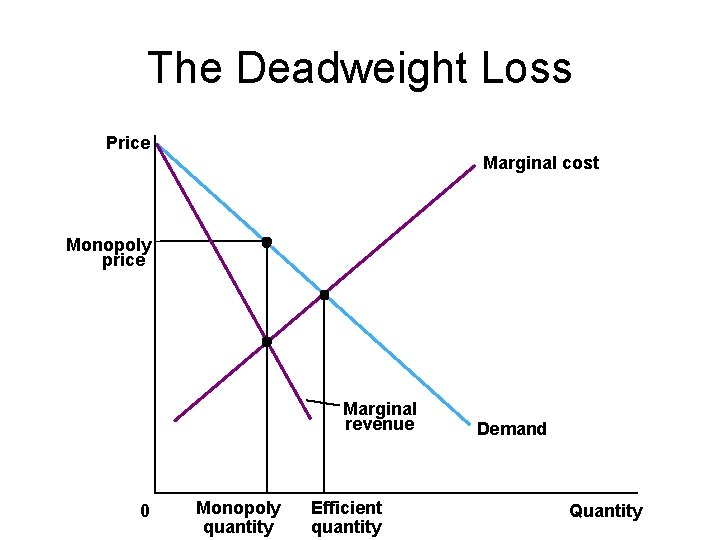The Deadweight Loss Price Marginal cost Monopoly price Marginal revenue 0 Monopoly quantity Efficient quantity Demand Quantity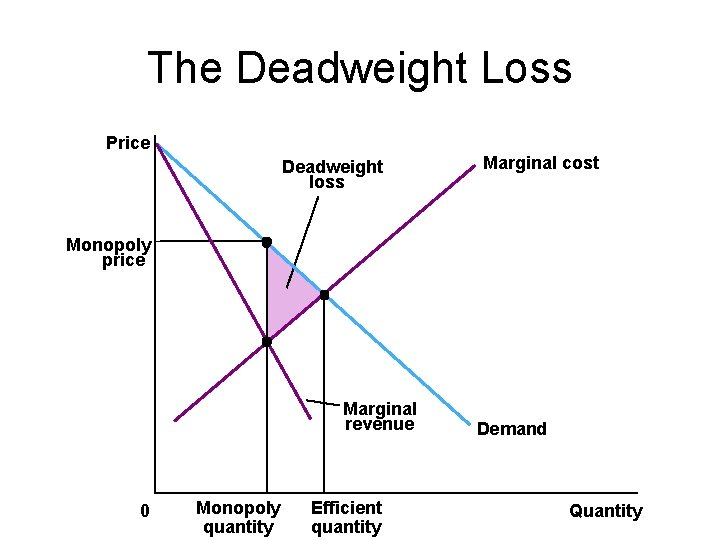The Deadweight Loss Price Deadweight loss Marginal cost Monopoly price Marginal revenue 0 Monopoly quantity Efficient quantity Demand Quantity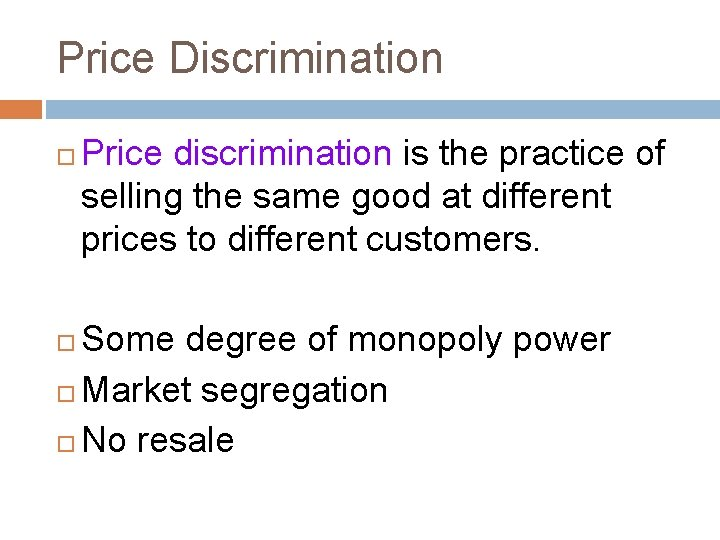Price Discrimination Price discrimination is the practice of selling the same good at different prices to different customers. Some degree of monopoly power Market segregation No resale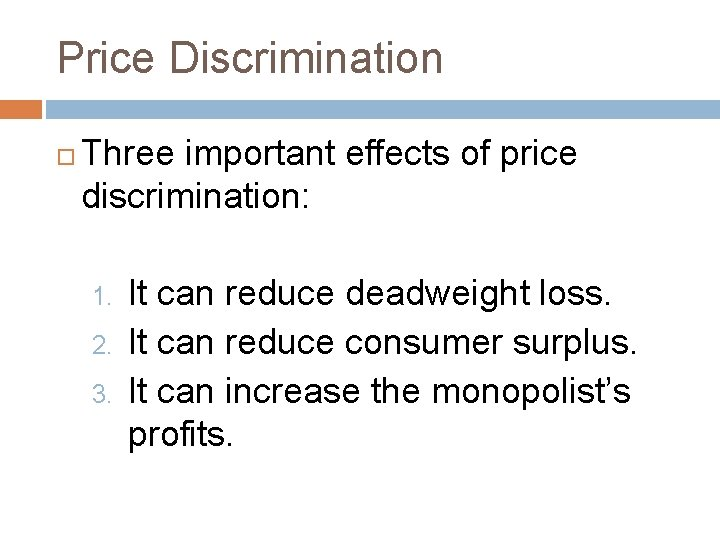Price Discrimination Three important effects of price discrimination: 1. 2. 3. It can reduce deadweight loss. It can reduce consumer surplus. It can increase the monopolist’s profits.Welfare Without Price Discrimination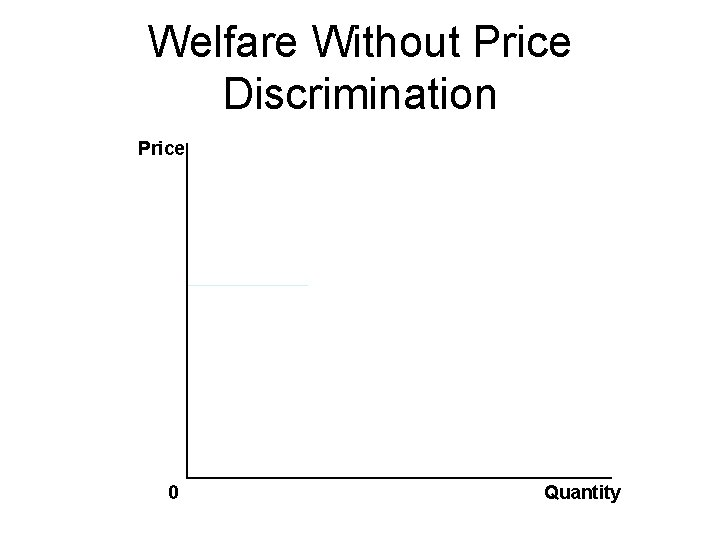Welfare Without Price Discrimination Price 0 QuantityWelfare Without Price Discrimination Price Consumer surplus Monopoly price Deadweight loss Profit Marginal cost Marginal revenue 0 Quantity sold Demand Quantity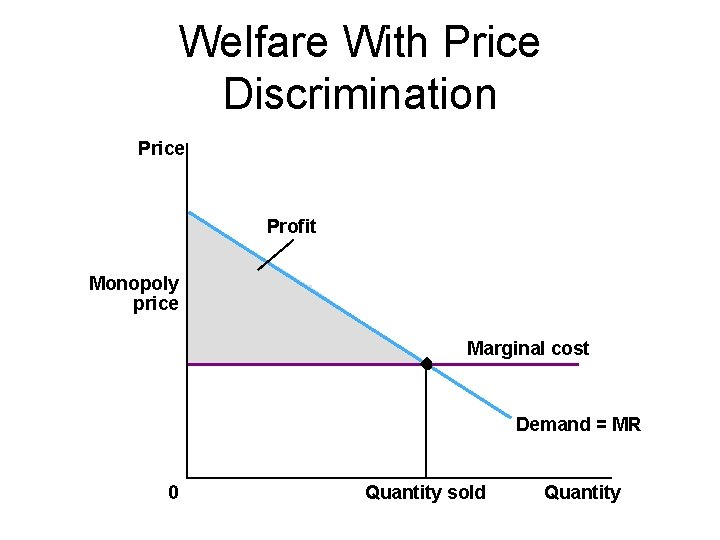Welfare With Price Discrimination Price Profit Monopoly price Marginal cost Demand = MR 0 Quantity sold Quantity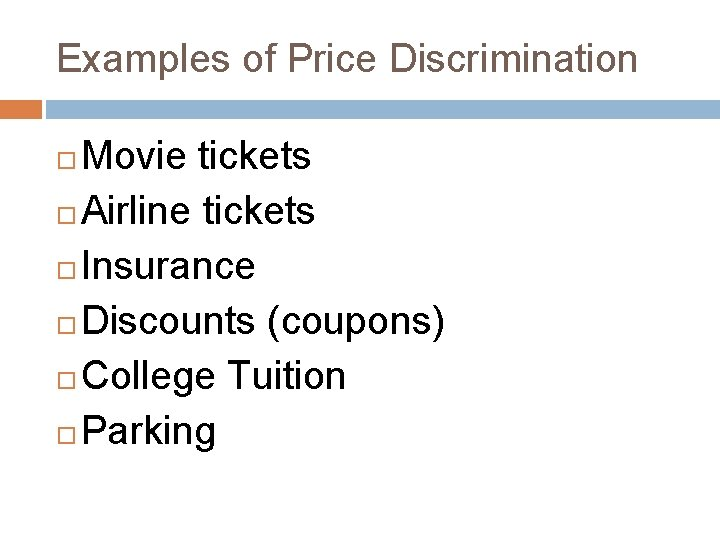Examples of Price Discrimination Movie tickets Airline tickets Insurance Discounts (coupons) College Tuition ParkingQuick Quiz! • Give two examples of price discrimination.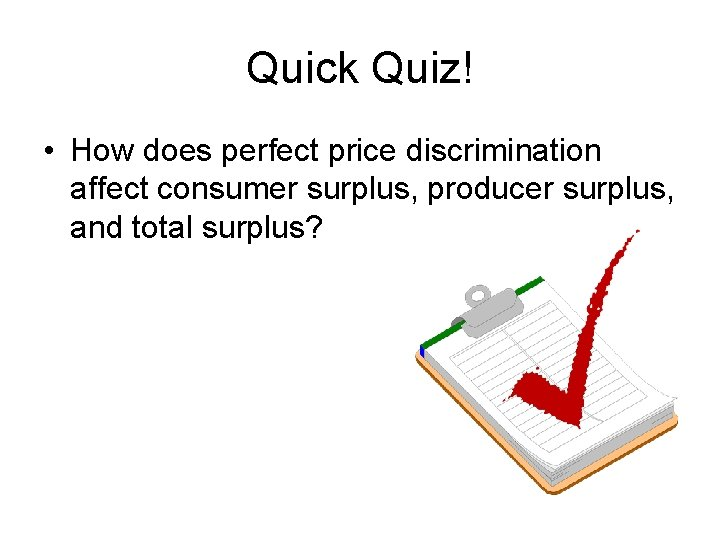Quick Quiz! • How does perfect price discrimination affect consumer surplus, producer surplus, and total surplus?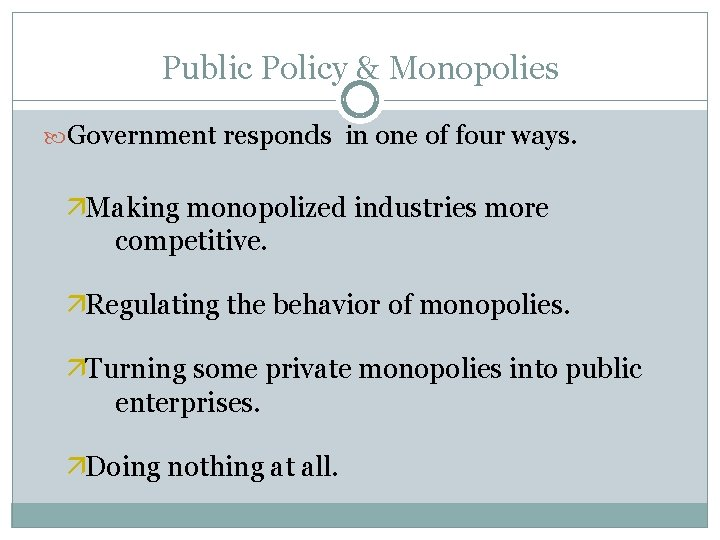Public Policy & Monopolies Government responds in one of four ways. äMaking monopolized industries more competitive. äRegulating the behavior of monopolies. äTurning some private monopolies into public enterprises. äDoing nothing at all.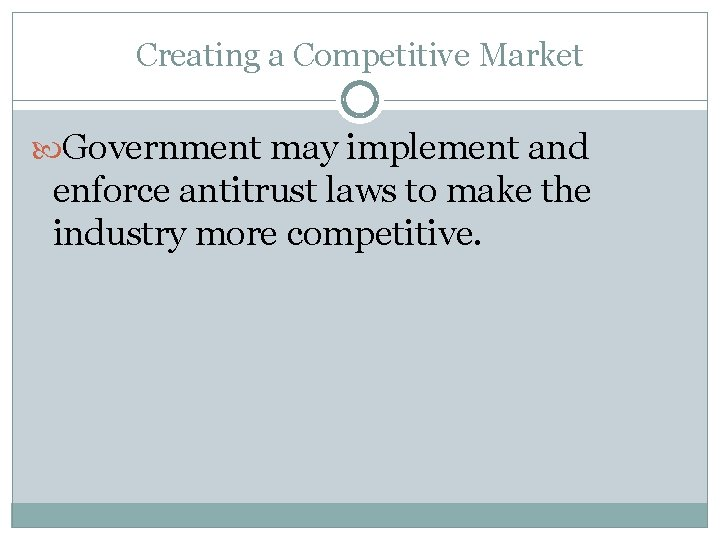Creating a Competitive Market Government may implement and enforce antitrust laws to make the industry more competitive.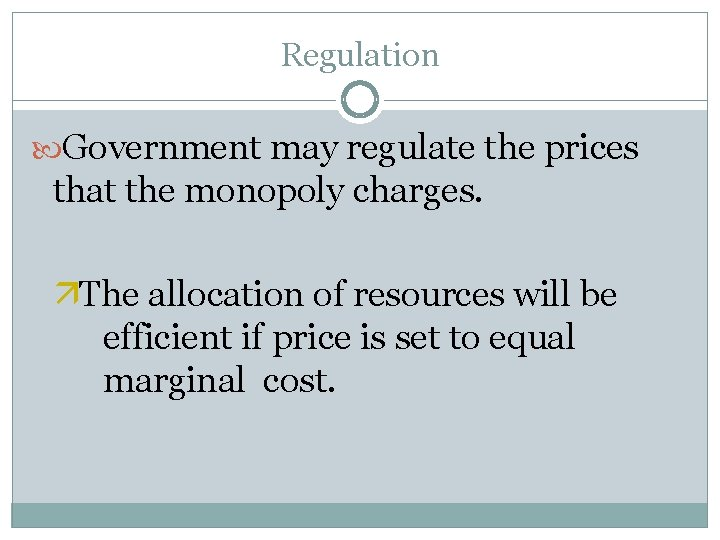Regulation Government may regulate the prices that the monopoly charges. äThe allocation of resources will be efficient if price is set to equal marginal cost.Regulation There are two practical problems with marginal-cost pricing. äPrice may be less than average total cost, and the firm will lose money. äIt gives the monopolist no incentive to reduce cost.Marginal-Cost Pricing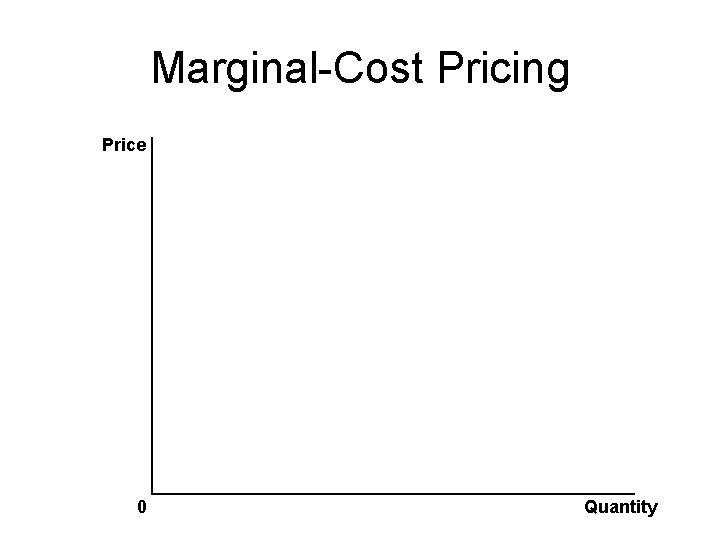Marginal-Cost Pricing Price 0 QuantityMarginal-Cost Pricing Price Average total cost Marginal cost Demand 0 Quantity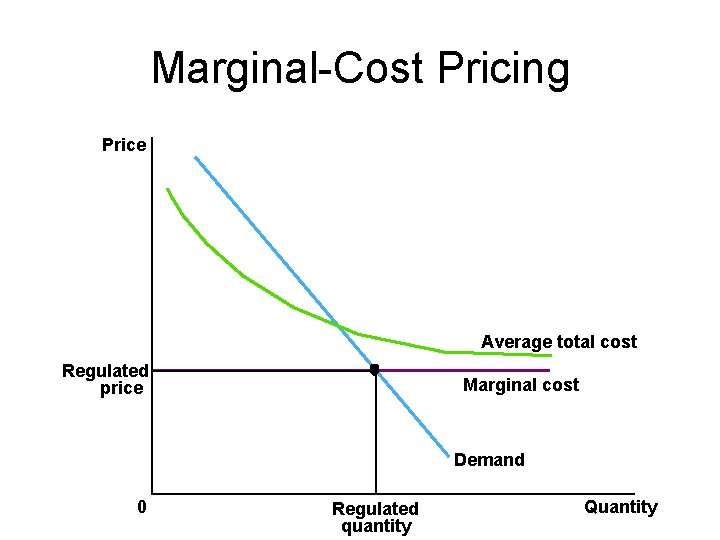Marginal-Cost Pricing Price Average total cost Regulated price Marginal cost Demand 0 Regulated quantity QuantityMarginal-Cost Pricing Price Average total cost Regulated price Average total cost Loss Marginal cost Demand 0 Regulated quantity Quantity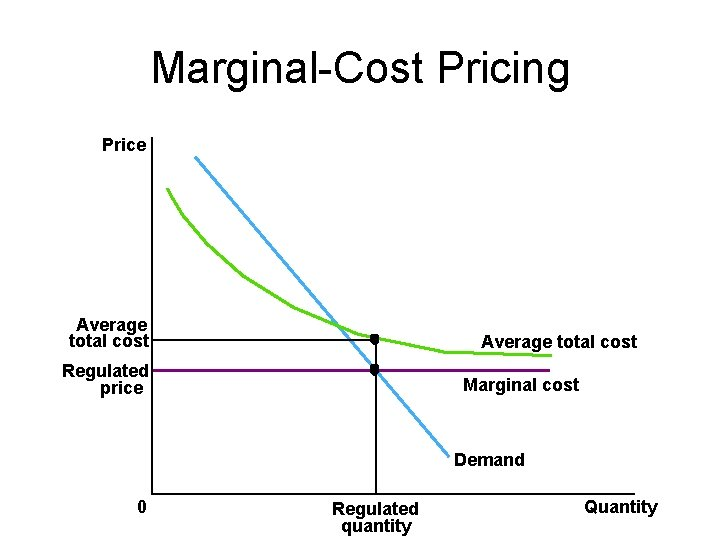Marginal-Cost Pricing Price Average total cost Regulated price Marginal cost Demand 0 Regulated quantity Quantity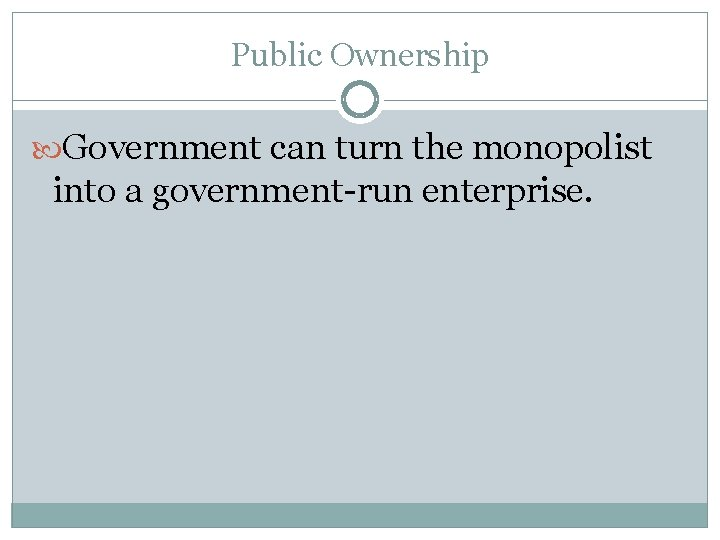Public Ownership Government can turn the monopolist into a government-run enterprise.Doing Nothing Government can do nothing at all if the market failure is deemed small compared to the imperfections of public policies.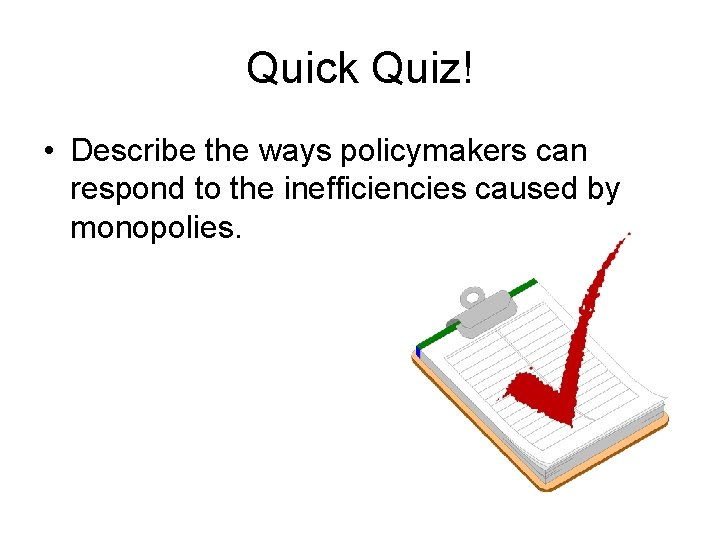Quick Quiz! • Describe the ways policymakers can respond to the inefficiencies caused by monopolies.Quick Quiz! • List a potential problem with each of these policy responses.Monopoly Misc o X-Inefficiency n o Rent Seeking Behavior n o o o Monopolies often operate at a higher cost than necessary Transfer wealth at someone else’s expense. Network Effect Allocative Efficiency (MC=D or P) Productive Efficiency (MC=ATC) Fair-Return/Cost of Service/Average Cost Pricing Marginal Cost PricingMarginal-Cost Pricing Price Average total cost Regulated price Marginal cost Demand 0 Regulated quantity QuantityThe Prevalence of Monopoly o How prevalent are the problems of monopolies? äMonopolies are around you……. äMost firms have some control over their prices because of differentiated products. äFirms with substantial monopoly power are rare. äFew goods are truly unique.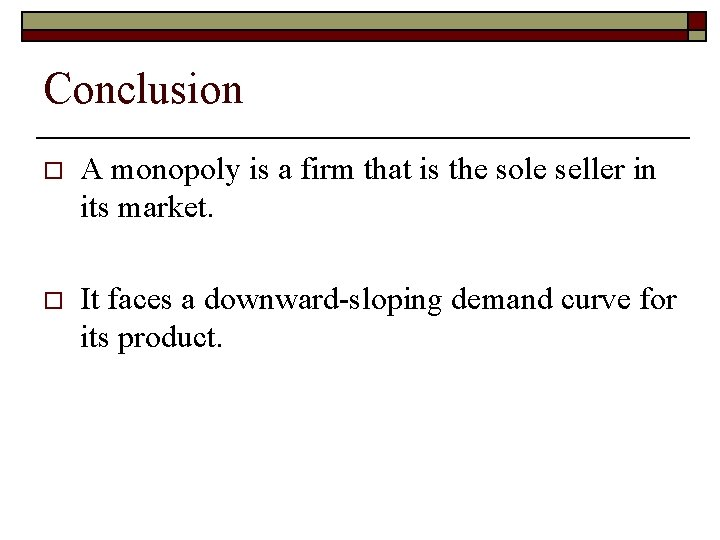Conclusion o A monopoly is a firm that is the sole seller in its market. o It faces a downward-sloping demand curve for its product.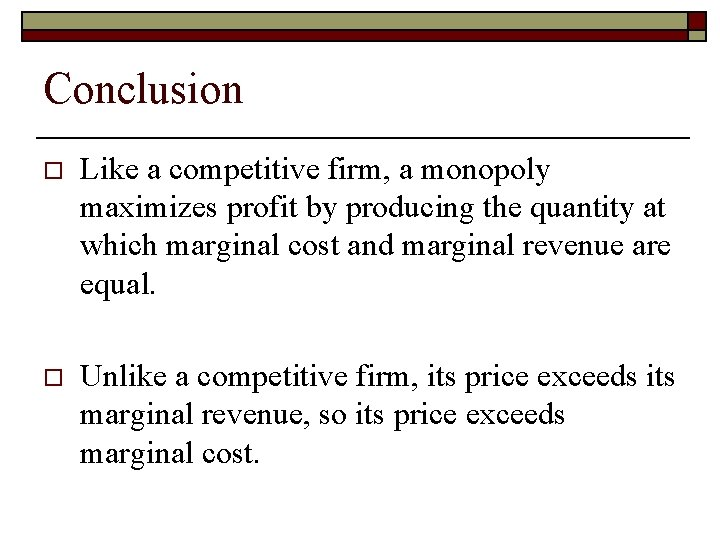Conclusion o Like a competitive firm, a monopoly maximizes profit by producing the quantity at which marginal cost and marginal revenue are equal. o Unlike a competitive firm, its price exceeds its marginal revenue, so its price exceeds marginal cost.Conclusion o A monopolist’s profit-maximizing level of output is below the level that maximizes the sum of consumer and producer surplus. o A monopoly causes deadweight losses similar to the deadweight losses caused by taxes.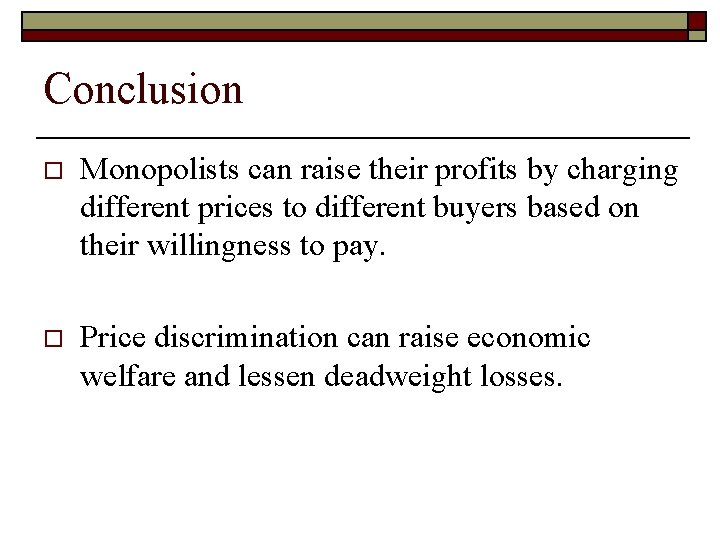Conclusion o Monopolists can raise their profits by charging different prices to different buyers based on their willingness to pay. o Price discrimination can raise economic welfare and lessen deadweight losses.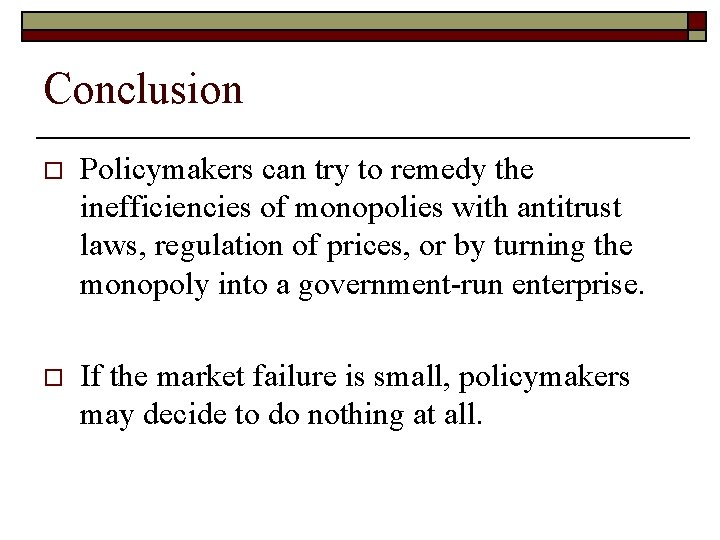Conclusion o Policymakers can try to remedy the inefficiencies of monopolies with antitrust laws, regulation of prices, or by turning the monopoly into a government-run enterprise. o If the market failure is small, policymakers may decide to do nothing at all.